# AP Statistics : Estimates

## Example Questions

### Example Question #1 : Estimates

What is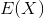, or the expected value offor any distribution?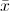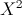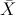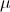Explanation:is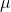, or the mean, of the population. This makes sense sinceliterally means the expected value of. The mean is the expected value ofExplanation:

### Example Question #1 : Estimates

The next local election is predicted to have a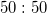split in votes for the top two candidates. How many people should be polled to obtain a margin of error of 3% at the 95% confidence level?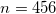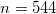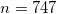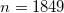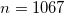Explanation:

If you remember the required formula, this problem is rather simple. Plug in the given numbers and simplify: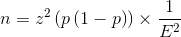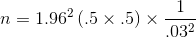If you don't remember the formula, this problem is more challenging. Your best bet in this case is to construct the confidence interval and rearrange to solve for the required sample size.

### Example Question #1 : Estimates

When dealing with confidence intervals, the margin of error gets smaller when z* gets ________ and n gets _________.

larger . . . smaller

There is no way to affect the margin of error in confidence intervals

smaller . . . larger

smaller . . . smaller

larger . . . larger

smaller . . . larger

Explanation:

For confidence intervals, a small margin of error is preferred, as it indicates that the parameter of interest has been narrowed down to a precise interval. Having a large sample population to work from as well as a small z* (z* gets smaller as the confidence level percent gets lower) can help obtain a small margin of error.

Explanation:

### Example Question #1 : Estimation